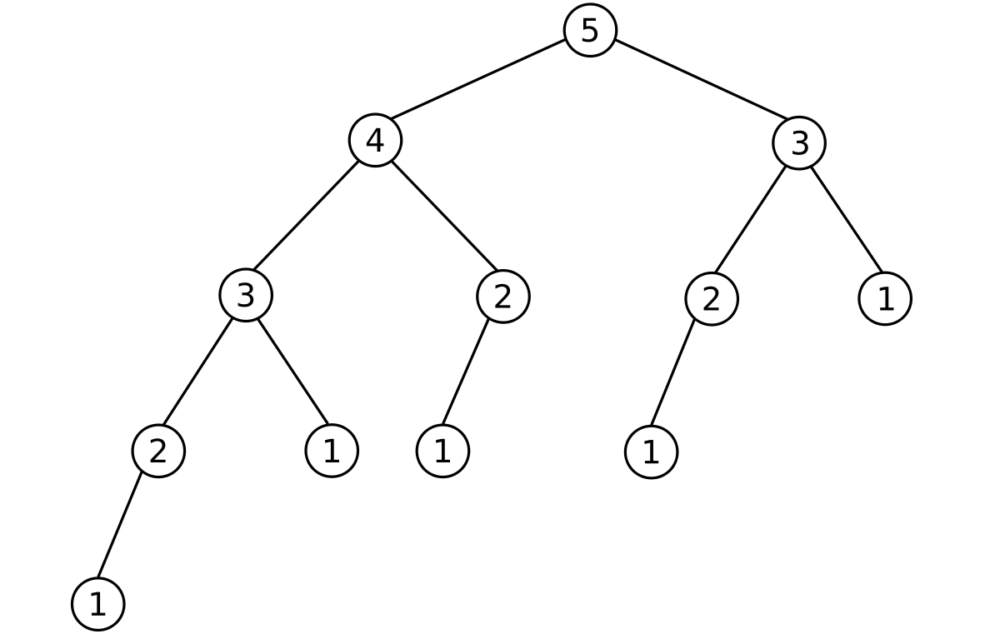# Circle Area and Fibonacci Calculation in JavaCalculating the area of a circle with a given radius.

``````package assignment1_donike;

public class CirlceArea {

public static void main(String[] args) {
// Defining double with Radius of circle
// Defining double that holds area, assigning Pi*(radius**2) using Math Method
//Print area within sentence and one line
}

} ``````

Calculating the area of a circle with a user give radius, catching errors caused by wrong input.

``````package assignment1_donike;
import java.io.BufferedReader; //using BR as instructed
import java.io.IOException; // No idea why i need to include that in line 18, but apparently I do

public class CircleAreaUserInput {

// creating new method that calculates area of circle from radius
// Defining double that holds area, assigning Pi*(radius**2) using Math Method
//Print area within sentence and one line
return area;
} // method

public static void main(String[] args) throws IOException{
// Creating boolean as condition for while loop
boolean valid_input = false;
// Creating user input var bc. it will be filled later within try/catch block and needs to be in the global scope

// Start loop, continue while valid input boolean == false
while(valid_input == false) {

// Creating Buffer
// print prompt to user in console
// read input line from console

// Try conversion to double, if successful set user_radius as converted double & valid_input=true and thus exit loop
try {
valid_input = true;}

// If conversion to double fails, give error message in console and keep in loop because valid_input is still false
catch(Exception e) {
System.out.println("Your input is not valid, please enter an integer, double or float\n\n");}

}// close while loop

// Pass user radius to method
System.out.println("The area of a circle with the radius " + user_radius + " is " + area_calc(user_radius));

} // close public static void main
} // close class

``````

Calculating the first 20 Fibonacci sequence numbers.

``````package assignment1_donike;

public class Fibonacci {

public static void main(String[] args) {

// starting points for additions are 0 and 1
int a = 0;
int b = 1;
// current will hold the value of the current addition of the two previous values and thus the Fibonacci number
int current = 0;
// counter will count iterations
int counter = 1;

while (counter<=20) {
// Addition of two previous numbers to get current Fib. value
current = a+b;
// Print counter of Fib. numbers and value at this position
System.out.println("Fibonacci Nr.\t"+counter+"\tis\t"+current+"\n");
// Change values to new positions: 	starting point (a) becomes what previously was 2nd summand
a = b;
// current Fib. value will become the 2nd summand in next iteration
b = current;
// increment counter + 1 to keep track of iterations
counter = counter+1;

} // close while loop

} //close public static void

} // close class
``````